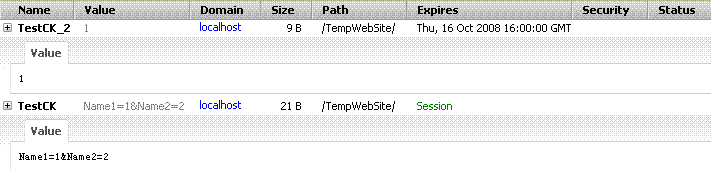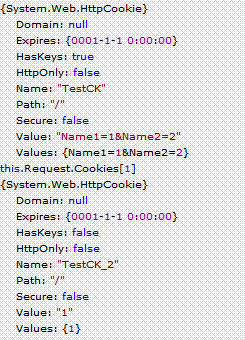expires属性——时间要转成GMT形式 ： toGMTString()；

domain属性

path属性

HttpOnly 属性限制了 cookie 对 HTTP 请求的作用范围。特别的，该属性指示用户代理忽略那些通过"非 HTTP" 方式对 cookie 的访问（比如浏览器暴露给js的接口）。注意 HttpOnly 属性和 Secure 属性相互独立：一个 cookie 既可以是 HttpOnly 的也可以有 Secure 属性。

Set-Cookie：customer=huangxp; path=/foo; domain=ibm.com; expires= Wednesday, 19-OCT-05 23:12:40 GMT; [secure];HttpOnly

expires:
"键/值"对: customer=huangxpcustomer=a1=huangxp&a2=huangxp

1,服务器端的操作

2,客户端操作

它们之间的交互我想通过下面的DEMO可以更好的让大家了解

protected void Page_Load(object sender, EventArgs e)
{
);
"Name1""1"
);
"Name2""2"
);

);
ck1.Value
= "1"
;

this

this
}

——————————————————————————————————————————————

}

= "TestCK=Name1=1&Name2=2"
;

var d1
= new Date(2008917
);
= "TestCK_2=1;expires=" +
d1;
}3, 对于在客户端设置的expires..这些属性,在服务器端获取不到,只能获取Value和Values属性(至于为什么会这样我也没有弄明白?)

Remove方法: 根据Key删除Values中的元素.
GetItemByKey方法: 根据Key获取元素
KeyValuePair类: 这个只是一个键/值对的类.

/* (Public)名称 */
this.Name = name;
this.KeyValues = new Array();

}

/* (Public)设置键/值对 */
Set : function(key, value){

switch(arguments.length){
case 0:
return;
break;
case 1:
if(!key){
return;
}

var item = this.GetItemByKey("__Default");
if(!item){
item = new KeyValuePair("__Default", key);
this.KeyValues.push(item);
}
else{
item.Value = key;
}
break;
case 2:
// key或value为空
if(!key || !value){
return;
}

var item = this.GetItemByKey(key);
//item为空的时候
if(!item){
item = new KeyValuePair(key, value);
this.KeyValues.push(item);
}
else{
item.Value = value;
}
break;
}

},

/* (Public)删除键 */
Remove : function(key){

//key为空
if(!key){
return;
}

var index = this._GetIndexByKey(key);

//存在数据
if(index > -1){
this.KeyValues.splice(index, 1);
}
},

/* (Public)获取值 */
Get : function(){
return this.GetItemByKey("__Default");
},

/* (Public)键/值对的索引 */
GetItemByKey : function(key){

//key为空
if(!key){
return;
}

//存在数据
if(this.KeyValues && this.KeyValues.length >0){
for(var i=0; i< this.KeyValues.length; i++){
var obj = this.KeyValues[i];

//关键字存在
if(obj.Key == key){
return obj;
break;
}
}
}

return null;
},

/* (Private)获取键/值对的Index */
_GetIndexByKey : function(key){

//存在数据
if(this.KeyValues && this.KeyValues.length >0){
for(var i=0; i< this.KeyValues.length; i++){
var obj = this.KeyValues[i];

//关键字存在
if(obj.Key == key){
return i;
break;
}
}
}

return -1;
}
}

/* 键/值对的类 */
function KeyValuePair(key, value){
this.Key = key;
this.Value = value;
}

}
}

}

if(arr == name){

break;
}
}
}

return null;
}

for(var i = 0; i< arrCookie.length; i++){
var str = "";

//存在键/值集合
if(obj.KeyValues){
for(var j =0; j< obj.KeyValues.length; j++){
var objKey = obj.KeyValues[j];

if(objKey.Key == "__Default"){
str += objKey.Value;
}
else{
str += objKey.Key + "=" + objKey.Value;
}

if(j != obj.KeyValues.length -1){
str += "&";
}
}

}

if(str){
document.cookie = obj.Name + "=" + str;
}
else{
}

if(expires){
}
if(domain){
}
if(path){
}
if(secure){
}
if(httponly){
}
}
}
}

if(arr.length > 1){

//存在键/值集合
var arrValues = strValue.split("&");
for(var j=0 ;j < arrValues.length; j++){
var arrKeyValue = arrValues[j].split("=");

if(arrKeyValue.length == 1){
}
else{
}
}

}
}©️2019 CSDN 皮肤主题: 大白 设计师: CSDN官方博客